﻿ MEMS 1×N Optical Switch Module
Hot products
•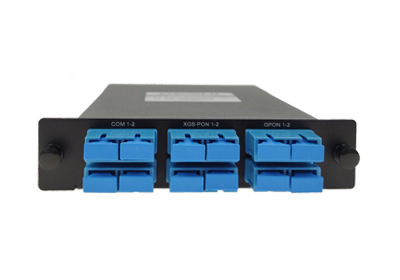••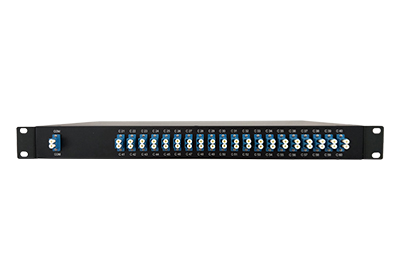•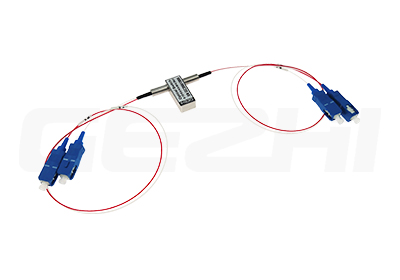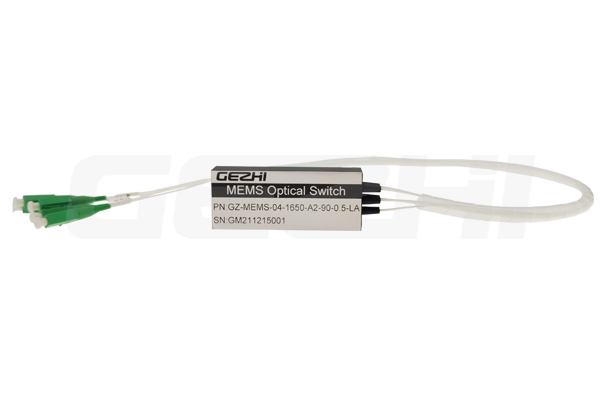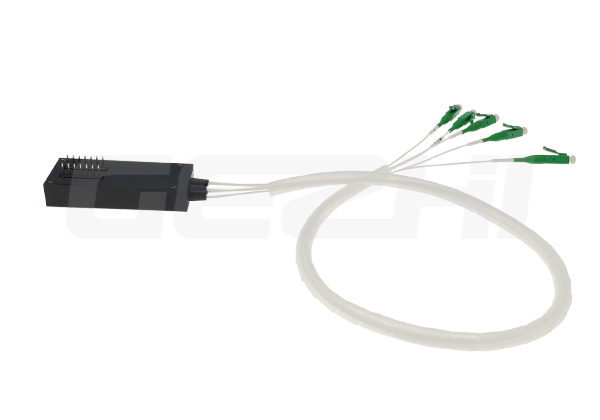MEMS 1×N Optical Switch Module
Based on MEMS technology. Compact size.
• Gezhi MEMS Optic Switches is easily integrated into optical systems.

MEMS technology is characterized by a long lifetime, high durability, and high reliability.

It is widely used in Telecom, Test & Measurement, Remote Sensing, Biomedical and other applications.

•  MEMS 1xN Optical Switch Module

Features

• Low Insertion loss

• Wide Wavelength Range

• Low Crosstalk

• High Stability, High Reliability

• Epoxy-free on Optical Path

• Latching and Non-latching

Applications

• Optical Network

• Protection/Restoration

• Optical Singnal Routing

• Network Test System

Technical Paremeter

 Parameters Unit MFSW-1×N (N≤16) Operating Wavelength nm 1260~1650 for SM, 850/1310 for MM Insertion Loss dB N≤8 ≤1.0 8

Ordering Information

 Type Channel Wavelength Fiber Type Fiber Diameter Fiber Length Connector GZ-MEMS 01=1x102=1x2.....16=1x16...... 85=850nm13=1310nm14=1490nm15=1550nm1625=1625nm1650=1650nm13/15=1310/1550nm 5=50/1256=62.5/1259=9/125X=Others 25=250um90=900um20=2.0mm30=3.0mmX=Others 1=1m2=1.5mX=Others 00=noneLP=LC/UPCLA=LC/APCSP=SC/UPCSA=SC/APCFP=FC/UPCFA=FC/APCMP=MPOX=Customized